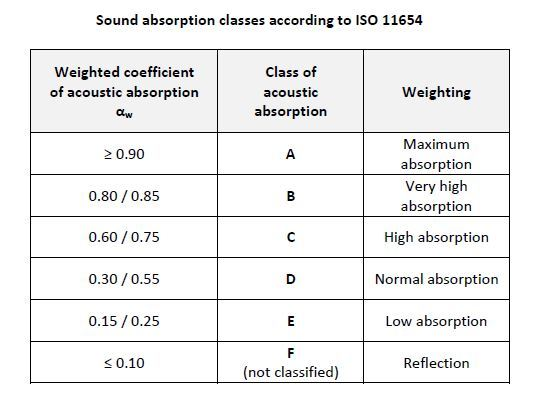Catálogo
Português

# Absorção acústics - ISO 11654NOISE ABSORPTION COEFFICIENTS ACCORDING TO ISO 11654 STANDARD
A wall in a common room is normally reverberant, that is, it does not have acoustic absorption properties and its weighted acoustic absorption coefficient is practically αw 0.
On the contrary, a 100% absorbing material has a coefficient αw 1.00.
The coefficients are measured by means of a reverberation room.

Coefficients
αs measures the frequency-dependent sound absorption in a reverberation room, it is used to measure the practical sound absorption coefficient αp.
αp is the practical acoustic absorption coefficient and it is frequency-related.
αw measures the weighted sound absorption regardless of frequency with classes going from A (maximum absorption) to F (reflection) to which the following indicators in brackets are also attributed:
- (L) if the material is efficient in the low frequency range, e.g. αw = 0.60 (L)
- (M) if the material is efficient in the mid-frequency range, e.g. αw = 0.70 (M)
- (H) if the material is efficient in the high frequency range, e.g. αw = 0.85 (H)
Combinations are possible, e.g. αw = 0.70 (MH) or αw = 0.60 (H).COPYRIGHT 2017 TODOS OS DIREITOS RESERVADOS - P.IVA/C.F. 00212670129 - CAP. SOC. € 1.150.000 - REG. IMP. Varese 00212670129 - REA VA-78719 - WEB DESIGN ZAKLAB